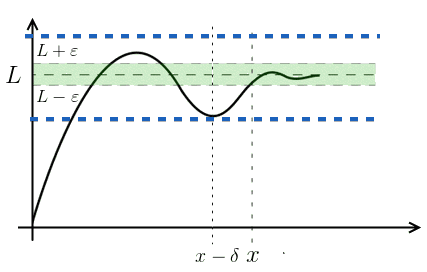ML Wiki

Limits

$\lim\limits_{x \to a} f(x) = L$

Definition

the limit of $f(x)$ as $x \to a$ is $L$:

• if $\forall \varepsilon > 0 \ \ \exists\, \delta > 0$ s.t. $x \ne a$ is within $\delta$ of $a$
• then $f(x)$ is within $\varepsilon$ of $L$

Interpretation

Interpretation:

• if as $x$ gets closer to $a$, $f(x)$ gets closer to $L$
• then $L$ is the limit of $f(x)$

or

• choose some output tolerance $\varepsilon$
• then there exists some input tolerance $\delta$ such that $L$ lies within $\varepsilon$ of $f(x)$
• if you change $\varepsilon$, you need to be able to update $\delta$ - this has to be true for any $\varepsilon$$x \to \infty$

• $a = \infty$ then
• $\lim\limits_{x \to \infty} f(x) = L$

What does it mean? Can think if it as of the "end" of the real line

Definition:

• the definition needs to be slightly adapted
• if $\forall \varepsilon > 0 \ \ \exists\, M > 0$ s.t. $|f(x) - L| < \varepsilon$ when $x > M$
• if there's no such $M$, then the limit does not exist

Interpretation: